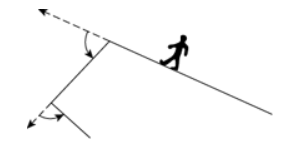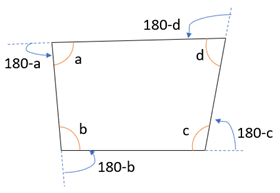Payal Tandon
Co-founder, e-GMAT
Welcome to e-GMAT Support!
I am Payal, Co-Founder of e-GMAT.
Feel free to ask any Query.We will be contacting you soon on

## OGQR 2020: Question No. 217A person walked completely around the edge of a park beginning at the midpoint of one edge and making the minimum number of turns, each with the minimum number of degrees necessary, as shown in the figure above. What is the sum of the degrees of all the turns that the person made?

1. One of the turns is 80 degrees.
2. The number of sides of the park is 4, all of the sides are straight, and each interior angle is less than 180 degrees.
 Source OGQR 2020 Type Data Sufficiency Topic Geometry Sub-Topic Polygons Difficulty Hard

### Solution

#### Steps 1 & 2: Understand Question and Draw Inferences

In this question, we are given that:

• A person is walking around the edge of a park.
• He starts walking from the midpoint of one edge of the park.
• The person will make the minimum number of turns with the minimum number of degrees

We need to find:

• The sum of the degrees of the turns made by the person.

To find the sum of degrees made by the person

• We need to find the angles measure on each turn
• And, we need to find how many turn the person takes
• Or, how many sides are there in the park.

With this understanding, let us now analyse the individual statements.

#### Step 3: Analyse Statement 1

“One of the turns is 80 degrees.”

From this statement, we cannot know how many sides does the park have neither the angles measure on each turn.

Hence, statement 1 is not sufficient to answer this question.

#### Step 4: Analyse Statement 2

The number of sides of the park is 4, all of the sides are straight, and each interior angle is less than 180 degrees

From this statement, we know that park has 4 sides.

• Hence, it is in the shape of quadrilateral with 4 sides as shown where a, b, c, and d are the internal angles of the quadrilateral.• The curved arrows show the turns taken by the person.
• Hence, the measure of angles in degrees on the turns are 180 – a, 180 – b, 180 – c, and 180 – d.
• Therefore, the sum of degrees of the turns taken by the person = 180 – a + 180 – b + 180 – c + 180 – d
• = 4 * 180 – (a + b + c + d)
• Now, a + b+ c + d = Sum of all the internal angles of the quadrilateral
• Per our conceptual understanding, the sum of the internal angles of a polygon having n sides = (n – 2) * 180
• Thus, a + b + c + d = (4 – 2) * 180 = 360
• Therefore, we can find the value of 4 * 180 – (a + b + c + d).

Hence, statement 2 is sufficient to find the answer.

#### Step 5: Combine Both Statements Together (If Needed)

Since we could determine the answer from statement 2 individually, this step is not required.

Hence, the correct answer is option B.#### Juhi Gupta

###### Browse Related Topics# Achieve 740+on the GMAT in 30 days!Sign up for our free trial and get400+ Practice questions with detailed solutions10+ hours of AI-driven video lessonsAdaptive mock test with ESR+ analysis